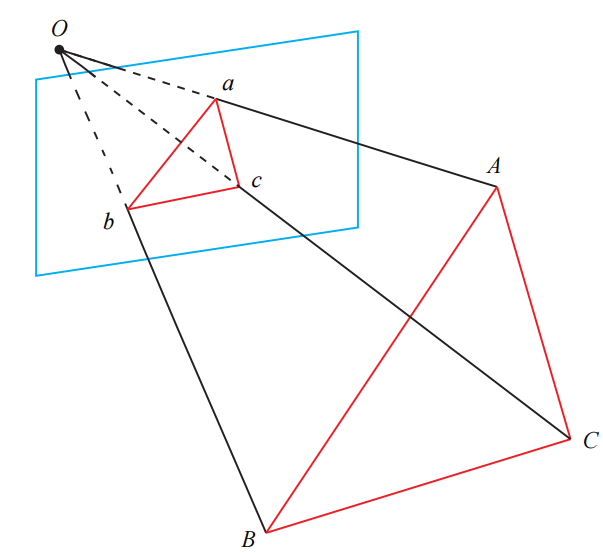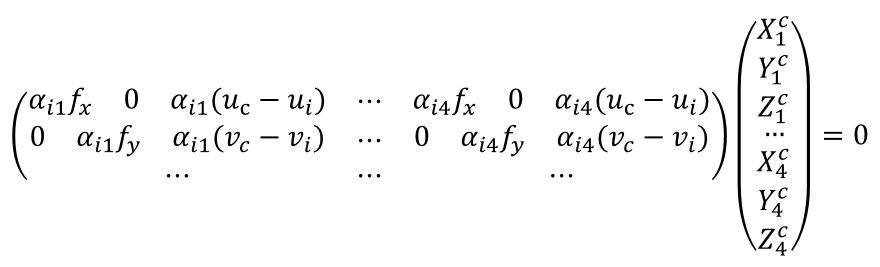0%

PNP即“Perspective-N-Points”，是求解 3D 到 2D 点对运动的方法。它描述了当我们知道n个3D空间点以及它们在图像上的位置时，如何估计相机所在的位姿。PnP 问题有很多种求解方法，例如用三对点估计位姿的 P3P（通常需要额外一个点进行验证结果），直接线性变换（DLT），EPnP（Efficient PnP，已知内参时用），UPnP（内参未知时用） 等等）。此外，还能用非线性优化的方式，构建最小二乘问题并迭代求解，也就是万金油式的 Bundle Adjustment

## P3P## EPnP

### 问题描述

EPnP即Efficient PnP，参考文献 EPnP: An Accurate O(n) Solution to the PnP Problem

• 特征点 $i$ 在世界坐标系的坐标$P_{i}^{w}=\left[\begin{array}{c}{x_{i}^{w}} \\ {y_{i}^{w}} \\ {z_{i}^{w}}\end{array}\right]$

• 特征点在成像平面上的坐标$p_{i}=\left[\begin{array}{l}{u_{i}} \\ {v_{i}}\end{array}\right]$

• 已知相机内参$K$

### 算法假设

EPnP的思想是无论世界系还是相机系下的$3D$点都可以由4个控制点线性组合，记：

• 世界系下4个控制点表示为:$\mathbf{c}_{j}^{w}, j=1, \cdots, 4$
• 相机系下4个控制点表示为:$\mathbf{c}_{j}^{c}, j=1, \cdots, 4$

EPnP算法将参考点的坐标表示为控制点坐标的加权和：

### 求解控制点在相机系下的坐标

$\left\{\mathbf{u}_{i}\right\}_{i=1, \cdots, n}$为相机下$3D$点$\left\{\mathbf{p}^c_{i}\right\}_{i=1, \cdots, n}$的图像坐标，则：